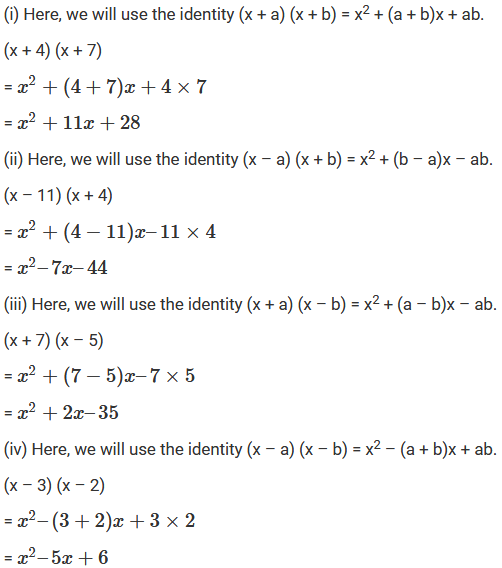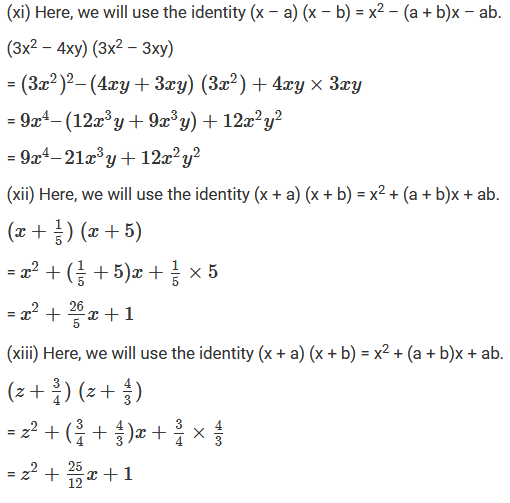×#### Thank you for registering.

One of our academic counsellors will contact you within 1 working day.

Click to Chat

1800-1023-196

+91-120-4616500

CART 0

• 0

MY CART (5)

Use Coupon: CART20 and get 20% off on all online Study Material

ITEM
DETAILS
MRP
DISCOUNT
FINAL PRICE
Total Price: Rs.

There are no items in this cart.
Continue Shopping• Complete JEE Main/Advanced Course and Test Series
• OFFERED PRICE: Rs. 15,900
• View Details

```Chapter 6: Algebraic Expressions and Identities Exercise – 6.7

Question: 1

Find the following products:

(i) (x + 4) (x + 7)

(ii) (x – 11) (x + 4)

(iii) (x + 7) (x – 5)

(iv) (x – 3) (x – 2)

(v) (y2 – 4) (y2 – 3)

(vi) (x + 4/3)(x + 3/4)

(vii) (3x + 5) (3x + 11)

(viii) (2x2 – 3) (2x2 + 5)

(ix) (z2 + 2) (z2 – 3)

(x) (3x – 4y) (2x – 4y)

(xi) (3x2 – 4xy) (3x2 – 3xy)

(xii) (x + 1/5)(x + 5)

(xiii) (z + 3/4)(z + 4/3)

(xiv) (x2 + 4) (x2 + 9)

(xv) (y2 + 12) (y2 + 6)

(xvi) (y2 + 5/7) (y2 – 14/5)

(xvii) (p2 + 16)(p2 – 14)

Solution:Question: 2

Evaluate the following:

(i) 102 x 106

(ii) 109 x 107

(iii) 35 x 37

(iv) 53 x 55

(v) 103 x 96

(vi) 34 x 36

(vii) 994 x 1006

Solution:

(i) Here, we will use the identity (x + a) (x + b) = x2 + (a + b)x + ab

102 x 106

= (100 + 2) (100 + 6)

= 1002 + (2 + 6)100 + 2 x 6

=10000 + 800 +12 = 10812

(ii) Here, we will use the identity (x + a) (x + b) = x2 + (a + b)x + ab

109 x 107

= (100 + 9) (100 + 7)

= 1002 + (9 + 7)100 + 9 x 7

=10000 + 1600 + 63 = 11663

(iii) Here, we will use the identity (x + a) (x + b) = x2 + (a + b)x + ab

35 x 37

= (30 + 5) (30 + 7)

= 302 + (5 + 7)30 + 5 x 7

= 900 + 360 + 35 = 1295

(iv) Here, we will use the identity (x + a) (x + b) = x2 + (a + b)x + ab

53 x 55

= (50 + 3) (50 + 5)

= 502 + (3 + 5)50 + 3 x 5

= 2500 + 400 + 15 = 2915

(v) Here, we will use the identity (x + a) (x – b) = x2 + (a – b)x – ab

103 x 96

= (100 + 3) (100 – 4)

= 1002 + (3 – 4)100 – 3 x 4

= 10000 – 100 – 12 = 9888

(vi) Here, we will use the identity (x + a) (x + b) = x2 + (a + b)x + ab

34 x 36

= (30 + 4) (30 + 6)

= 302 + (4 + 6)30 + 4 x 6

= 900 + 300 + 24 = 1224

(vii) Here, we will use the identity (x – a) (x + b) = x2 + (b – a)x – ab

994 x 1006

= (1000 – 6) x (1000 + 6)

= 10002 + (6 – 6) x 1000 – 6 x 6

= 1000000 – 36 = 999964
```### Course Features

• 728 Video Lectures
• Revision Notes
• Previous Year Papers
• Mind Map
• Study Planner
• NCERT Solutions
• Discussion Forum
• Test paper with Video Solution